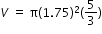# Sample Test Item PreviewExport Print
General Information
Related Benchmark: MAFS.8.G.3.9
Reporting Category: Geometry
Type: EE: Equation Editor
Difficulty: N/A
Question:

A water container in the shape of a cone has a height of 5 inches and a diameter of 3.5 inches.

Enter an equation in the first blank box and a number in the second blank box to complete the statements about the water container.

A. The formula to calculate the container's volume, V, with the given measurements is ________.

B. The container can hold approximately _____ cubic inches of water. Round your answer to the nearest hundredth.

A.(or any equivalent equation)